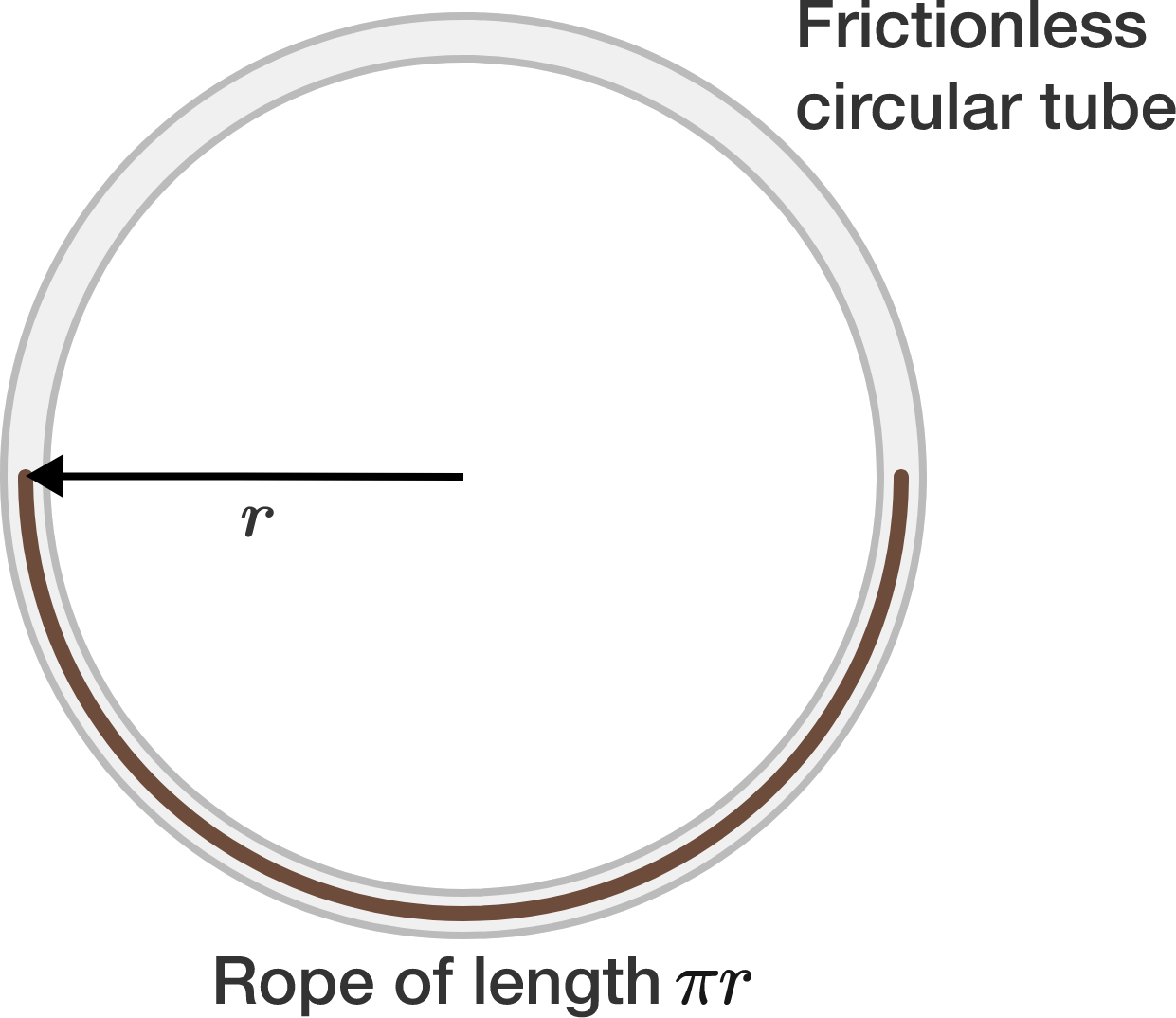# Rope in a circular tubeA rope of mass $M$ having length $\pi r$ is placed in a vertical circular tube centered at the origin. The mean radius of the tube is $r = \SI{\dfrac{20}{\pi^{3}}}{\meter}$. The width of the tube is such that it just fits the rope.

The rope is slightly pulled from one end and released to oscillate. If the tube is frictionless, find the time period (in seconds) of the small oscillations. $\big($Take acceleration due to gravity $g=\SI[per-mode=symbol]{10}{\meter\per\second\squared}.\big)$

×